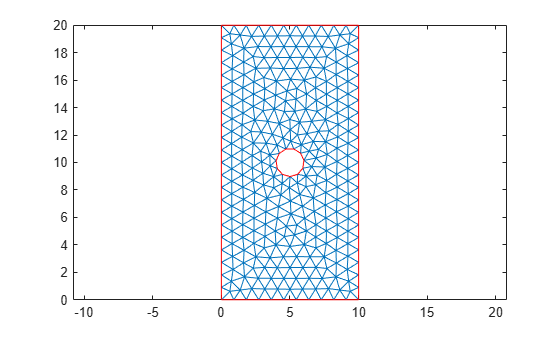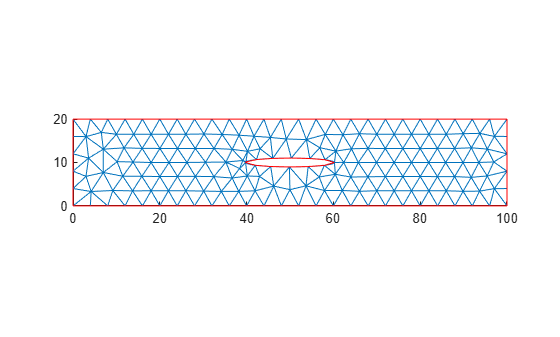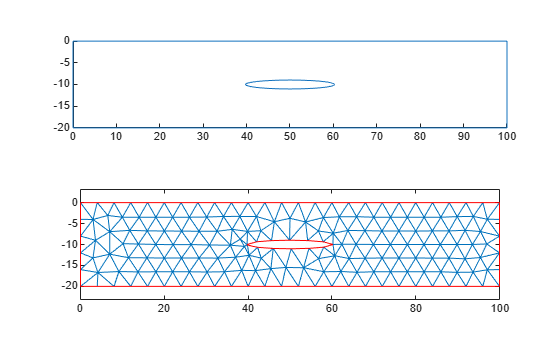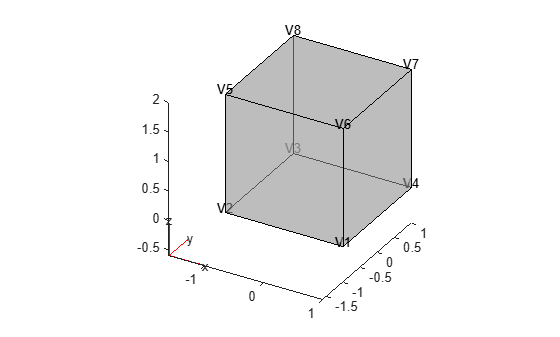scale

Scale geometry

Description

example

scale(g,s) scales the geometry g by the factor s with respect to the origin.

example

scale(g,s,refpoint) scales the geometry with respect to the reference point refpoint.

h = scale(g,___) returns a handle h to the resulting geometry object g. Use this syntax with any input arguments from the previous syntaxes.

If the original geometry is a DiscreteGeometry object, then the function modifies the original geometry and returns the handle h to the modified DiscreteGeometry geometry object. If the original geometry is an AnalyticGeometry object, then h is a handle to a new DiscreteGeometry geometry object. In this case, the original geometry remains unchanged.

Examples

collapse all

Scale a 2-D geometry along the x- and y-axis and ensure consistency with the mesh.

Create a model.

model = createpde;

Import and plot a geometry.

g = importGeometry(model,'PlateHolePlanar.stl');
pdegplot(model)Mesh the geometry and plot the mesh.

generateMesh(model);

figure
pdemesh(model)Scale the geometry by a factor of 10 along the x-axis.

scale(g,[10 1])
ans =
DiscreteGeometry with properties:

NumCells: 0
NumFaces: 1
NumEdges: 5
NumVertices: 5
Vertices: [5x3 double]

Plot the geometry.

figure
pdegplot(model)Plot the geometry and mesh. The scale function modifies a geometry, but it does not modify a mesh.

figure
pdegplot(model)
hold on
pdemesh(model)After modifying the geometry, always regenerate the mesh.

generateMesh(model);

figure
pdegplot(model)
hold on
pdemesh(model)Reflect the geometry across the x-axis and regenerate the mesh.

scale(g,[1 -1]);
generateMesh(model);

Plot the resulting geometry and mesh.

figure
subplot(2,1,1)
pdegplot(model)
subplot(2,1,2)
pdemesh(model)Enlarge a geometry: first uniformly in all directions, and then using different scaling factors along different axes.

Create and plot a geometry.

g = multicuboid(1,1,1);
pdegplot(g,'VertexLabels','on','FaceAlpha',0.5)Scale the geometry by a factor of 2 uniformly along all coordinate axes. Plot the result.

scale(g,2);
pdegplot(g,'VertexLabels','on','FaceAlpha',0.5)Now scale by factors of 2, 3, and 4 along the x-, y-, and z-axes, respectively. Plot the result.

scale(g,[2 3 4]);
pdegplot(g,'VertexLabels','on','FaceAlpha',0.5)Flip the geometry upside down by scaling it with the factor -1 and using the bottom front corner (vertex 1) as a reference point.

scale(g,[1 1 -1], [2 -3 0]);
pdegplot(g,'VertexLabels','on','FaceAlpha',0.5)Input Arguments

collapse all

Geometry, specified as a DiscreteGeometry or AnalyticGeometry object.

Scaling factor, specified as a real number or vector of two or three real numbers. Use one value for uniform scaling in all directions. Use a vector of two or three elements to specify different scaling factors along the x-, y-, and, for a 3-D geometry, z-axes.

Reference point for scaling specified as a vector of two or three real numbers for a 2-D and 3-D geometry, respectively

Output Arguments

collapse all

Resulting geometry, returned as a handle. If the original geometry g is a DiscreteGeometry object, then h is a handle to the modified DiscreteGeometry geometry object g. If g is an AnalyticGeometry object, then h is a handle to a new DiscreteGeometry geometry object. In this case, the original geometry g remains unchanged.

Tips

• scale modifies a geometry, but it does not modify a mesh. After modifying a geometry, regenerate the mesh to ensure a proper mesh association with the new geometry.

• If the scaling factor is negative, then the coordinates will flip their signs. The scaling factor of -1 mirrors the existing geometry if the reference point is the origin.

• If g is an AnalyticGeometry object, and you want to replace it with the resulting discrete geometry, assign the output to the original geometry, for example, g = scale(g,20).